Author: Oscar Cronquist Article last updated on September 28, 2022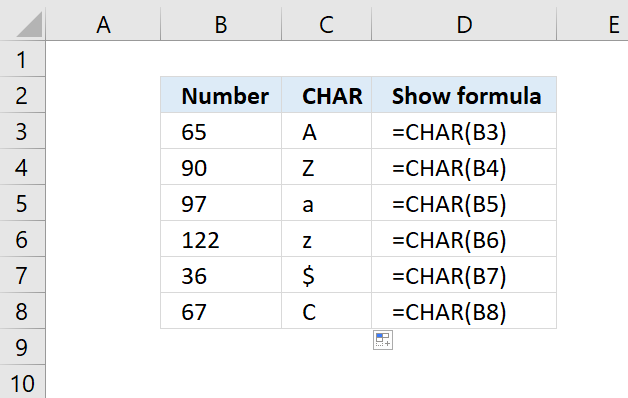Use the CHAR function to convert a number to the corresponding character. This is determined by your computer's character set.

 Windows ANSI Macintosh Macintosh character set

CHAR(text)

## 2. CHAR Function Arguments

 text Required. The number for which you want the corresponding character.

## 3. CHAR Function example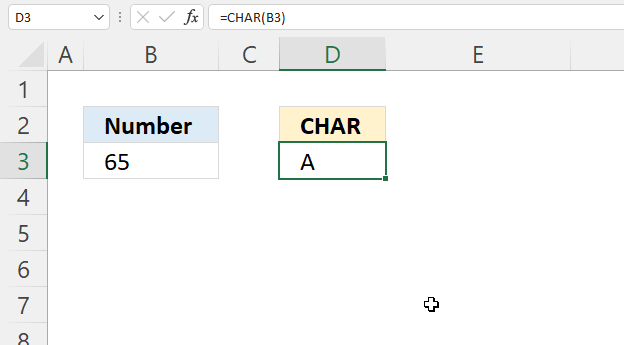The image above shows how to convert a number to a character using the CHAR function.

Formula in cell D3:

=CHAR(B3)

### Explaining formula

The CHAR function converts a number between 1 and 255 to a corresponding character.

CHAR(B3)

becomes

CHAR(65)

and returns "A".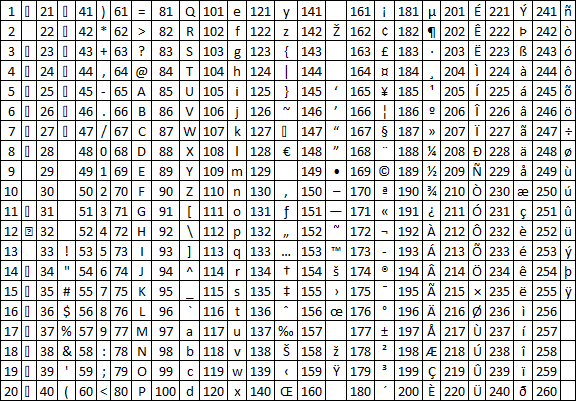The image above shows numbers and corresponding characters.

## 4. How to convert comma delimiting numbers to a string of characters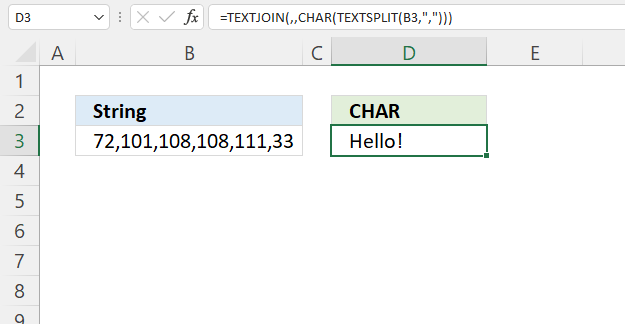This example demonstrates a formula that converts a series of numbers to characters based on the ANSI character set. The TEXTSPLIT function is a new Excel 365 function.

Formula in cell D3:

=TEXTJOIN(, , CHAR(TEXTSPLIT(B3, ",")))

### Explaining formula

#### Step 1 - Split numbers using a comma as a delimiting character

The TEXTSPLIT function lets you split a string into an array across columns and rows based on delimiting characters.

TEXTSPLIT(Input_Textcol_delimiter, [row_delimiter], [Ignore_Empty])

TEXTSPLIT(B3, ",")

becomes

TEXTSPLIT("72,101,108,108,111,33", ",")

and returns

{"72","101","108","108","111","33"}.

There is no need to convert "text" numbers to regular numbers, the CHAR function accepts "text" numbers.

#### Step 2 - Convert numbers in array to characters

CHAR(TEXTSPLIT(B3, ","))

becomes

CHAR({"72","101","108","108","111","33"})

and returns

{"H","e","l","l","o","!"}

#### Step 3 - Join characters

The TEXTJOIN function allows you to combine text strings from multiple cell ranges and also use delimiting characters if you want.

TEXTJOIN(delimiterignore_emptytext1[text2], ...)

TEXTJOIN(, , CHAR(TEXTSPLIT(B3, ",")))

becomes

TEXTJOIN(, , {"H","e","l","l","o","!"})

and returns "Hello!".

## 5. How to list all characters based on ANSI code

Number 65 to 90 correspond to A to Z. Number 97 to 122 correspond to a to z.

 2  34 " 66 B 98 130 ‚ 162 194 Â 226 â 3  35 # 67 C 99 131 ƒ 163 195 Ã 227 ã 4  36 \$ 68 D 100 132 „ 164 196 Ä 228 ä 5  37 % 69 E 101 133 … 165 197 Å 229 å 6  38 & 70 F 102 134 † 166 198 Æ 230 æ 7  39 ' 71 G 103 135 ‡ 167 199 Ç 231 ç 8  40 ( 72 H 104 136 ˆ 168 200 È 232 è 9 41 ) 73 I 105 137 ‰ 169 201 É 233 é 10 42 * 74 J 106 138 Š 170 202 Ê 234 ê 11 43 + 75 K 107 139 ‹ 171 203 Ë 235 ë 12 44 , 76 L 108 140 Œ 172 204 Ì 236 ì 13 45 - 77 M 109 141  173 205 Í 237 í 14  46 . 78 N 110 142 Ž 174 206 Î 238 î 15  47 / 79 O 111 143  175 207 Ï 239 ï 16  48 0 80 P 112 144  176 208 Ð 240 ð 17  49 1 81 Q 113 145 ‘ 177 209 Ñ 241 ñ 18  50 2 82 R 114 146 ’ 178 210 Ò 242 ò 19  51 3 83 S 115 147 “ 179 211 Ó 243 ó 20  52 4 84 T 116 148 ” 180 212 Ô 244 ô 21  53 5 85 U 117 149 • 181 213 Õ 245 õ 22  54 6 86 V 118 150 – 182 214 Ö 246 ö 23  55 7 87 W 119 151 — 183 215 × 247 ÷ 24  56 8 88 X 120 152 ˜ 184 216 Ø 248 ø 25  57 9 89 Y 121 153 ™ 185 217 Ù 249 ù 26  58 : 90 Z 122 154 š 186 218 Ú 250 ú 27  59 ; 91 [ 123 155 › 187 219 Û 251 û 28 60 < 92 \ 124 156 œ 188 220 Ü 252 ü 29 61 = 93 ] 125 157  189 221 Ý 253 ý 30 62 > 94 ^ 126 158 ž 190 222 Þ 254 þ 31 63 ? 95 _ 127 159 Ÿ 191 223 ß 255 ÿ 32 64 @ 96 ` 128 160 192 224 à 256

Here is how to create a table containing all characters returned from char using numbers 1 to 255 in Excel:

=CHAR(SEQUENCE(32,8))

### 5.1 Explaining formula

#### Step 1 - Create numbers from 1 to 255

The SEQUENCE function creates a list of sequential numbers.

SEQUENCE(rows, [columns], [start], [step])

SEQUENCE(32, 8)

returns

{1, 2, 3, 4, 5, 6, 7, 8; 9, ... , 256}

#### Step 2 - Return characters

CHAR(SEQUENCE(32, 8))

returns the table above without the numbers.

## 6. How to identify a new line character in an Excel formula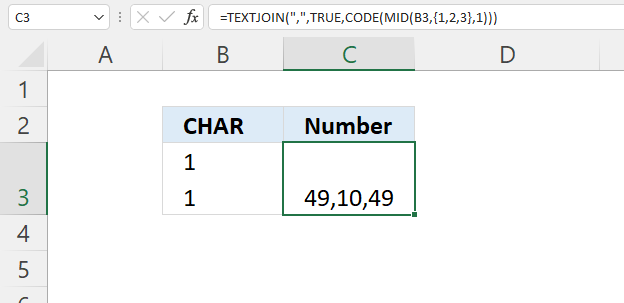The image above shows a formula that converts characters to ANSI code. Cell B3 contains a carriage return between 1 and 1. The number for a new line or carriage return is 10.

I used the following formula to identify the numbers for each character in cell B3.

Formula in cell C3:

=TEXTJOIN(",", TRUE, CODE(MID(B3, {1, 2, 3}, 1)))

You can use CHAR(10) to create a new line in a formula, see the next image below.

### 6.1 Explaining formula

#### Step 1 - Create an array

Cell B3 contains three characters. To extract each character we need an array containing numbers from 1 to 3.

The curly brackets and delimiting characters let you create an array containing constants.

{1,2,3}

#### Step 2 - Split text string

The MID function returns a substring from a string based on the starting position and the number of characters you want to extract.

MID(textstart_numnum_chars)

MID(B3,{1,2,3},1)

becomes

MID("1
1",{1,2,3},1)

and returns

{"1","
","1"}.

#### Step 3 - Calculate the number for each character

The CODE function returns a specific number for the first character of the text argument, determined by your computer's character set.

CODE(text)

CODE(MID(B3,{1,2,3},1))

becomes

CODE({"1","
","1"})

and returns {49, 10, 49}.

#### Step 4 - Join numbers

The TEXTJOIN function allows you to combine text strings from multiple cell ranges and also use delimiting characters.

TEXTJOIN(",",TRUE,CODE(MID(B3,{1,2,3},1)))

becomes

TEXTJOIN(",",TRUE,{49, 10, 49})

and returns 49,10,49.

## 7. How to create a new line in an Excel formula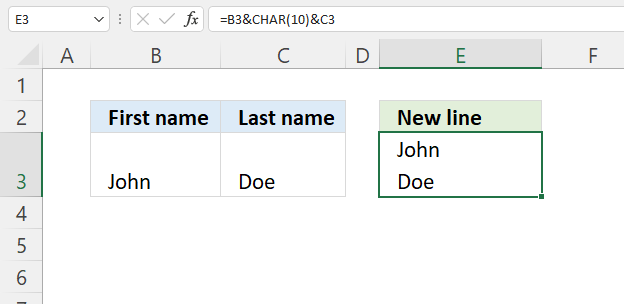The image above shows how to create a line break in a formula, cell E3 contains a formula that concatenates strings in cells B3 and C3 and inserts a line break in between.

Formula in cell E3:

=B3&CHAR(10)&C3

### 7.1 Explaining formula

#### Step 1 - Create a new line

The following function and number 10 create a new line.

CHAR(10)

#### Step 2 - Concatenate text

The ampersand character concatenates strings in an Excel formula.

B3&CHAR(10)&C3

returns

John
Doe

## 8. How to show a new line in a cell (wrap text)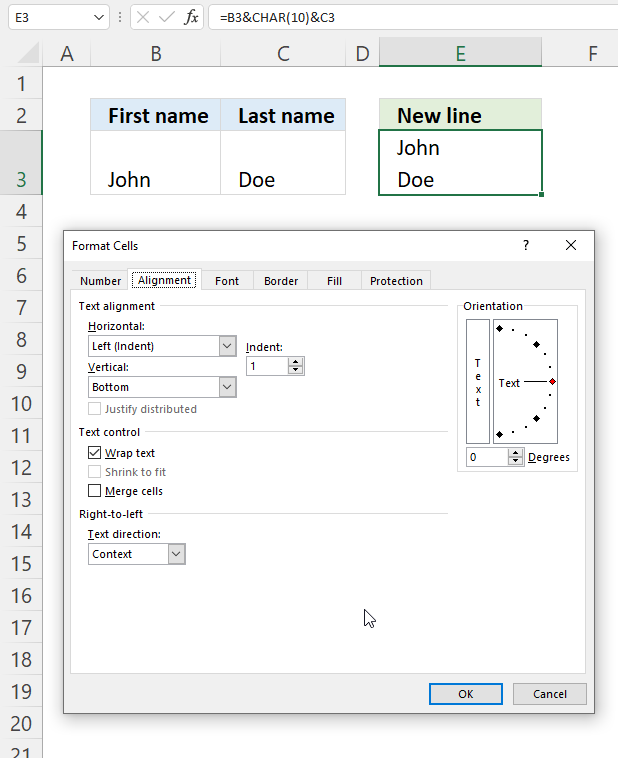You need to allow the text to wrap in a cell, here is how to do that:

1. Select the cell.
2. Press CTRL + 1, and the "Format Cells" dialog box appears.
3. Press with mouse on tab "Alignment, see the image above.
4. Press with left mouse button on check box "Wrap text" to enable it.
5. Press with left mouse button on OK button.

## 9. How to create a tab in an Excel formula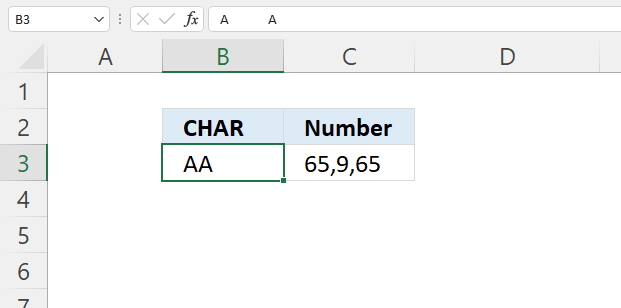The picture above shows that the character is CHAR(9), however, the cell doesn't display the tab. Note that the formula bar shows the tab.

Formula in cell C3:

=TEXTJOIN(",", TRUE, CODE(MID(B3, {1, 2, 3}, 1)))

I am using the same formulas as in section 5 to identify the corresponding numbers for each character and concatenate tab characters with given strings.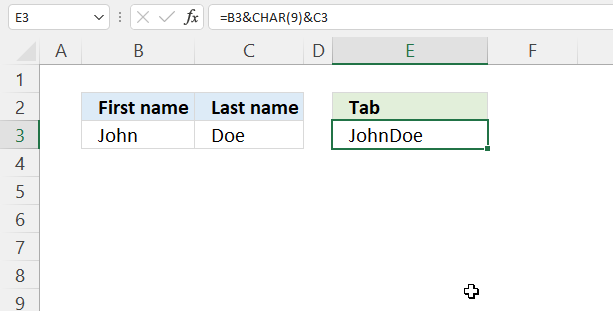Formula in cell E3:

=B3&CHAR(9)&C3

## 8. How to create superscript characters in an Excel formula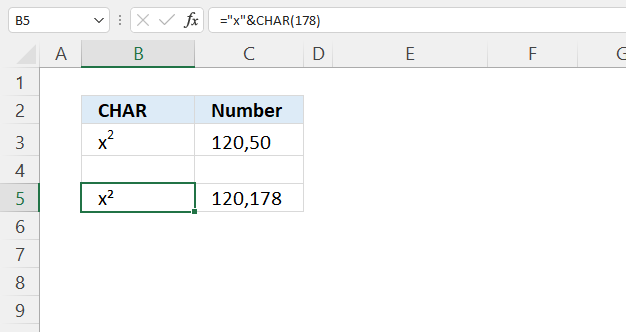The formula in cell B5 demonstrates how to use superscript characters, there are not that many you can use.

Formula in cell B5:

=B3&CHAR(178)&C3

The CHAR function lets you use a couple of superscript characters see list below, and the UNICHAR function gives you more options.

 174 x® 175 x¯ 176 x° 177 x± 178 x² 179 x³ 180 x´